6. Examples.¶

6.1. Interference examples.¶

6.1.1. Young’s experiment.¶

from LightPipes import *
import matplotlib.pyplot as plt
import matplotlib.image as mpimg

"""
Young's experiment.
Two holes with radii, R, separated by, d, in a screen are illuminated by a plane wave. The interference pattern
at a distance, z, behind the screen is calculated.
"""

wavelength=5*um
size=20.0*mm
N=500
z=50*cm
R=0.3*mm
d=1.2*mm

plt.imshow(img); plt.axis('off')

plt.show()F=Begin(size,wavelength,N)
F1=CircAperture(R/2.0,-d/2.0, 0, F)
F2=CircAperture(R/2.0, d/2.0, 0, F)
F=BeamMix(F1,F2)
F=Fresnel(z,F)
I=Intensity(2,F)
plt.imshow(I, cmap='rainbow'); plt.axis('off');plt.title('intensity pattern')
plt.show()

(png, hires.png, pdf)6.1.2. Michelson interferometer.¶

#! /usr/bin/env python
from LightPipes import *
import matplotlib.pyplot as plt
import matplotlib.image as mpimg

wavelength=632.8*nm #wavelength of HeNe laser
size=10*mm # size of the grid
N=300 # number (NxN) of grid pixels
R=3*mm # laser beam radius
z1=8*cm # length of arm 1
z2=7*cm # length of arm 2
z3=3*cm # distance laser to beamsplitter
z4=5*cm # distance beamsplitter to screen
Rbs=0.5 # reflection beam splitter
tx=1*mrad; ty=0.0*mrad # tilt of mirror 1
f=50*cm # focal length of positive lens

plt.imshow(img); plt.axis('off')
plt.show()#Generate a weak converging laser beam using a weak positive lens:
F=Begin(size,wavelength,N)
F=GaussHermite(0,0,1,R,F)
F=Lens(f,0,0,F)

#Propagate to the beamsplitter:
F=Forvard(z3,F)

#Split the beam and propagate to mirror #2:
F2=IntAttenuator(1-Rbs,F)
F2=Forvard(z2,F2)

#Introduce tilt and propagate back to the beamsplitter:
F2=Tilt(tx,ty,F2)
F2=Forvard(z2,F2)
F2=IntAttenuator(Rbs,F2)

#Split off the second beam and propagate to- and back from the mirror #1:
F10=IntAttenuator(Rbs,F)
F1=Forvard(z1*2,F10)
F1=IntAttenuator(1-Rbs,F1)

#Recombine the two beams and propagate to the screen:
F=BeamMix(F1,F2)
F=Forvard(z4,F)
I=Intensity(1,F)

plt.imshow(I,cmap='jet'); plt.axis('off');plt.title('intensity pattern')
plt.show()

(png, hires.png, pdf)6.2. Diffraction examples.¶

6.2.1. Diffraction from a circular aperture.¶

from LightPipes import *
import matplotlib.pyplot as plt

wavelength=1*um
size=25.0*mm
N=1000

F=Begin(size,wavelength,N)
F=CircAperture(5*mm, 0, 0, F)
F=Forvard(100*cm,F)
I=Intensity(0,F)

plt.imshow(I,cmap='jet')
plt.show()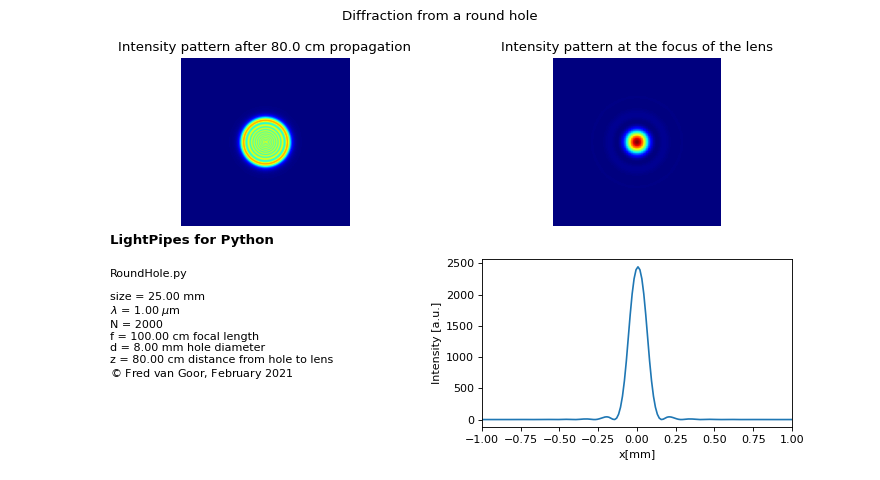6.2.2. Spot of Poisson.¶

from LightPipes import *
import matplotlib.pyplot as plt

wavelength=5*um
size=25.0*mm
N=500

F=Begin(size,wavelength,N)
F=GaussHermite(0,0,1,size/3,F)
F=CircScreen(3*mm,0*mm,0*mm,F)
F=Fresnel(20*cm,F)
I=Intensity(2,F)

plt.imshow(I,cmap='jet'); plt.axis('off'); plt.title("Poisson's spot")
plt.show()6.3. Non-diffractive Bessel beam.¶

A Bessel beam has the interesting property that it does not diffract and that it keeps its shape over large distances. Several meters, depending on parameters, can be realized. Applications of Bessel beams take advantage of the very large size of the focus, which cannot be obtained using lenses or mirrors. For example generation of a long narrow plasma channel can be realized using a high-power laser beam converted into a Bessel beam by an axicon lens.

Besides an axicon, a combination of an annular slit and a positive lens or concave mirror can be used in staed. In the following example a Poisson spot is generated by illuminating a circular disk by a plane mono-chromatic beam. The disk is positioned in the primary focus of a positive lens so that the waves originating from the edge of teh disk will be collimated. By blocking the rest of the incoming beam only the edge waves remain which results in a non-diffracting Bessel beam.

reference: J.Durnin, “Exact solutions for nondiffracting beams. I. The scalar theory.” JOSA A, Vol. 4, Issue 4, pp. 651-654 (1987)

6.3.1. From Poisson spot to a non-diffractive Bessel beam.¶

The waves originating from Huygens point-sources at the edge of the disk can be considered as a collection of spherical waves which are all in phase because the disk is illuminated by a monochromatic plane wave. Each spherical wave has the same amplitude as well. As a result these waves interfere constructively to a Poisson spot near the axis. It can be shown that the intensity distribution is approximately given by:

$$I(r,z) \approx I_0 J_0 ^2 ( \frac{2 \pi \alpha r}{ \lambda } )$$

with:

$$\alpha = \frac{a}{r}$$ is the angle of the wavefront near the axis, $$2a$$ is the diameter of the disk

The width of the beam is given by:

$$w(z)=\frac{2.44}{ \pi } \frac{ \lambda z}{a}$$

and is proportional to the distance, z.

from LightPipes import *
import matplotlib.pyplot as plt
import matplotlib.image as mpimg
import math
import numpy as np

plt.imshow(img); plt.axis('off');plt.title('Spot of Poisson')
plt.show()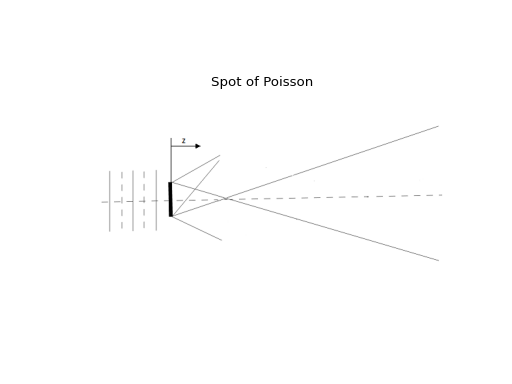wavelength=1000.0*nm
size=10*mm
N=1000

N2=int(N/2)
ZoomFactor=10
NZ=N2/ZoomFactor

a=1.5*mm
z_start=0.001*cm; z_end= 150*cm;
steps=11;
delta_z=(z_end-z_start)/steps
z=z_start
#x=[]
#for i in range(1,N):
#   x.append((-size/2 + i*size/N)/mm)

F=Begin(size,wavelength,N);
F=GaussHermite(0,0,1,size/3.5,F)
F=CircScreen(a,0,0,F)

w0=2.44/math.pi*wavelength/a
for i in range(1,steps):
w=w0*z

#y=[]
F=Fresnel(delta_z,F);
I=Intensity(0,F);
#for j in range(1,N):
#    y.append(I[N2][j])
plt.subplot(2,5,i)

s='z= %3.1f m \n' % (z/m) + 'width = %3.2f mm' % (w/mm)
plt.title(s, fontsize=8)
#plt.plot(x,y)
plt.imshow(I,cmap='jet');plt.axis('off')
plt.axis([N2-NZ, N2+NZ, N2-NZ, N2+NZ])
#plt.axis([-1, 1, 0,1])
z=z+delta_z
plt.show()

(png, hires.png, pdf)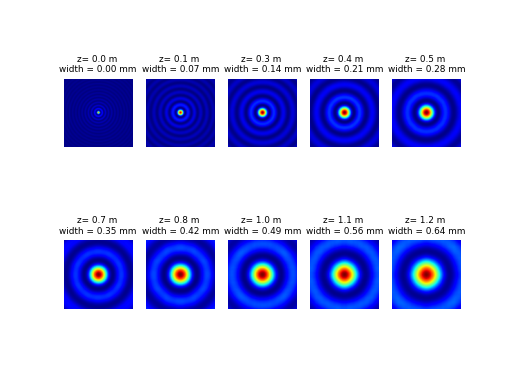6.3.1.1. Collimating the edge waves with a lens.¶

When the disk is placed in the primary focus of a postive lens,the spherical waves are collimated and the angle, $$\alpha$$ remains constant. As a result the beam does not diverge anymore. Ofcourse the light passing the disk at larger distances from the edge will be focussed as well by the lens and will disturb the Bessel beam. This light can easily been blocked with an extra aperture surrounding the disk resulting in an annular slit.

from LightPipes import *
import matplotlib.pyplot as plt
import matplotlib.image as mpimg

plt.imshow(img); plt.axis('off');plt.title('Spot of Poisson -> Bessel beam with lens')
plt.show()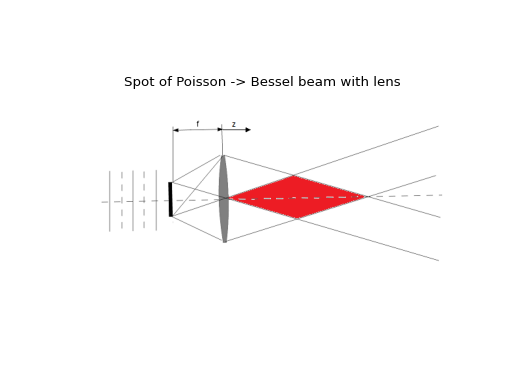wavelength=1000.0*nm
size=10*mm
N=1000

N2=int(N/2)
ZoomFactor=10
NZ=N2/ZoomFactor

a=1.5*mm
f=500*mm
z_start=0.001*cm; z_end= 150*cm;
steps=11;
delta_z=(z_end-z_start)/steps
z=z_start

F=Begin(size,wavelength,N);
F=GaussHermite(0,0,1,size/3.5,F)
F=CircScreen(a,0,0,F)
F=Fresnel(f,F);
F=Lens(f,0,0,F);
for i in range(1,steps):
F=Fresnel(delta_z,F);
I=Intensity(0,F);
plt.subplot(2,5,i)
s='z= %3.1f m' % (z/m)
plt.title(s)
plt.imshow(I,cmap='jet');plt.axis('off')
plt.axis([N2-NZ, N2+NZ, N2-NZ, N2+NZ])
z=z+delta_z
plt.show()

(png, hires.png, pdf)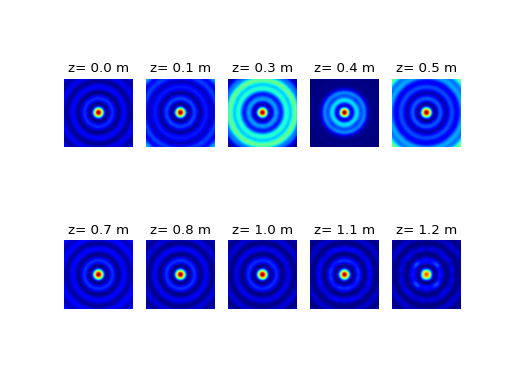6.3.2. Generation of a Bessel beam with a lens and an annular slit.¶

By positioning an aperture around the disk a non-difracting Bessel beam is generated over a distance given by the ‘overlap area’. From geometric optics this distance can be estimated by:

$$z_{max}=\frac{Df}{a}$$, where $$D$$ and $$f$$ are the diameter and focallength of the lens respectively.

from LightPipes import *
import matplotlib.pyplot as plt
import matplotlib.image as mpimg

plt.imshow(img); plt.axis('off');plt.title('Bessel beam with annular slit')
plt.show()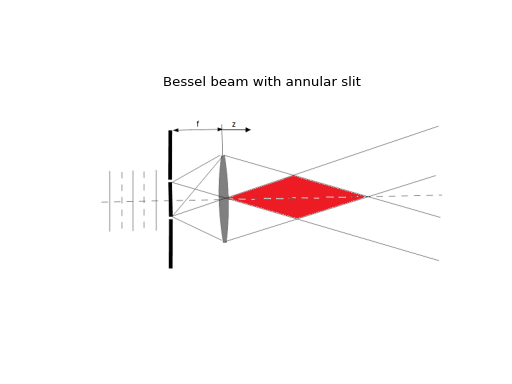wavelength=1000.0*nm
size=10*mm
N=1000

N2=int(N/2)
ZoomFactor=10
NZ=N2/ZoomFactor

a=1.5*mm
f=500*mm
z_start=0.001*cm; z_end= 150*cm;
steps=11;
delta_z=(z_end-z_start)/steps
z=z_start

F=Begin(size,wavelength,N);
F=GaussHermite(0,0,1,size/3.5,F)
F=CircScreen(a,0,0,F)
F=CircAperture(a+0.1*mm,0,0,F)
F=Fresnel(f,F);
F=Lens(f,0,0,F);
for i in range(1,steps):
F=Fresnel(delta_z,F);
I=Intensity(0,F);
plt.subplot(2,5,i)
s='z= %3.1f m' % (z/m)
plt.title(s)
plt.imshow(I,cmap='jet');plt.axis('off')
plt.axis([N2-NZ, N2+NZ, N2-NZ, N2+NZ])
z=z+delta_z
plt.show()

(png, hires.png, pdf)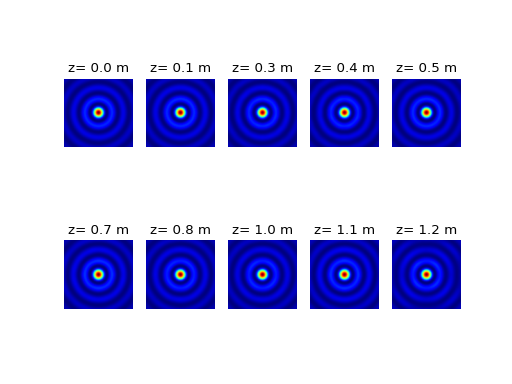6.3.3. Generation of a Bessel beam with an axicon.¶

Using an annular slit is of course not very efficient because most of the incoming (laser) beam is not used. A much more efficient way to generate a Bessel beam is the use of an axicon. With such an element in principle all the incoming light is converted. Although all the light is converted most applications are only interacting with the central lobe of the beam, which only carries a small fraction of the total beam power.

from LightPipes import *
import matplotlib.pyplot as plt
import matplotlib.image as mpimg

plt.imshow(img); plt.axis('off');plt.title('Bessel beam with axicon')
plt.show()wavelength=1000.0*nm
size=10*mm
N=1000

N2=int(N/2)
ZoomFactor=10
NZ=N2/ZoomFactor

phi=179.7/180*3.1415; n1=1.5
z_start=0.001*cm; z_end= 150*cm;
steps=11;
delta_z=(z_end-z_start)/steps
z=z_start

F=Begin(size,wavelength,N);
F=GaussHermite(0,0,1,size/3.5,F)
F=Axicon(phi,n1,0,0,F)

for i in range(1,steps):
F=Fresnel(delta_z,F);
I=Intensity(0,F);
plt.subplot(2,5,i)
s='z= %3.1f m' % (z/m)
plt.title(s)
plt.imshow(I,cmap='jet');plt.axis('off')
plt.axis([N2-NZ, N2+NZ, N2-NZ, N2+NZ])
z=z+delta_z
plt.show()

(png, hires.png, pdf)6.4. Laser examples.¶

6.4.1. Laser simulation, stable laser resonator.¶

A typical laser consists of two (concave or convex) mirrors separated some distance and a gain medium mostly based on stimulated emission. One (or both) of the mirrors is partly transmissive and when the mirrors are well aligned and the losses are below some maximum the radiation field inside the resonator will grow starting from noise by spontaneous emission as a function of the number of round trips. The laser intensity will be structured in a number of resonator modes depending on the wavelength, the curvatures of and the distance between the mirrors and especially the diameter of an intra-cavity aperture.Laser resonator with gain.

In the python script below a number of parameters can be adjusted which allows the study of several important features of a laser. In the movie we show Q-switching to generate short high intensity pulses by changing the reflectivity of the outcoupling mirror, operation on high-order transversal modes by opening the aperture, changing the resonator g-parameters to study the stability criterion, injection of a high-order Gauss-Hermite mode and the effect of thin wires inside the resonator.

laser_simulation.py
#!/usr/bin/env python
import matplotlib
matplotlib.use("TkAgg")
from matplotlib.backends.backend_tkagg import FigureCanvasTkAgg
import matplotlib.pyplot as plt
import math
import time
import sys
if sys.version_info < 3:
from Tkinter import *
import Tkinter as Tk
else:
from tkinter import *
import tkinter as Tk
from LightPipes import *

root = Tk.Tk()
root.wm_title("Laser with stable resonator")
root.wm_protocol("WM_DELETE_WINDOW", root.quit)
power=[]
roundtrip=0

wavelength=10600*nm;
size=20*mm;
N=100; N2=int(N/2)
Isat=131*W/cm/cm; alpha=0.0067/cm; Lgain=30*cm;

f1=2.0*m
f2=5*m

L=30*cm
T=1;
Reflect=0.9;
w0=2.4*mm
n=10;
xwire=10.0*mm
ywire=10.0*mm
dt=2*L/2.998*1e-8
fig=plt.figure(figsize=(6,9))

canvas = FigureCanvasTkAgg(fig, master=root)
canvas._tkcanvas.pack(side=Tk.LEFT, fill=Tk.BOTH, expand=1)
v=StringVar()
F=Begin(size,wavelength,N)

def TheExample():
#start_time=time.time()
global F,f1,f2,L,w0,roundtrip
global Reflect
w0=float(scale_w0.get())*mm/2
xwire=float(scalexwire.get())*mm
ywire=float(scaleywire.get())*mm
f1=float(scale_f1.get()*cm)/2
f2=float(scale_f2.get()*cm)/2
L=float(scale_L.get())*cm
Reflect=float(scale_Reflect.get())
alpha=float(scale_gain.get())/cm
F=RandomIntensity(time.time(),1e-8,F)
F=CircAperture(w0,0,0,F)
F=RectScreen(size,0.2*mm,0.0,ywire,0.0,F)
F=RectScreen(0.2*mm,size,xwire,0.0,0.0,F)
Iw=Intensity(0,F)
F=Lens(f2,0,0,F);
F=Forvard(L,F); F=Gain(Isat,alpha,Lgain,F);
F=Lens(f1,0,0,F);
F=Tilt(tx,ty,F)
F=Forvard(L,F); F=Gain(Isat,alpha,Lgain,F);
F=IntAttenuator(Reflect,F)
P=Power(F)*(1-Reflect)*size/N*size/N
power.append(P); roundtrip=roundtrip+1

if (roundtrip>500):
power.pop(0)
Iout=Isat*(alpha*Lgain-0.5*math.log(1/Reflect))*math.pi*w0*w0

ax1.clear()
ax2.clear()

g1=1-L/(2*f1);
g2=1-L/(2*f2);
g=g1*g2
v.set(  "Power=%5.3f W\n"% P+
"g1 = %5.3f\n"%g1+
"g2 = %5.3f\n"%g2+
"g  = %5.3f\n"%g
)
ax1.imshow(Iw,cmap='rainbow'); ax1.axis('off'); ax1.axis('equal')
ax1.set_title('laser mode')
ax2.plot(power); ax2.set_ylim(0,10); ax2.set_xlim(0,500)
s='%3.1f ns/div'% (2.0*L/2.988*1000.0)
ax2.set_xlabel(s); ax2.set_ylabel('power [W]')
ax2.tick_params(
axis='x',          # changes apply to the x-axis
which='both',      # both major and minor ticks are affected
bottom='on',      # ticks along the bottom edge are off
top='on',         # ticks along the top edge are off
labelbottom='off')
ax2.grid()
canvas.show()
#print("Execution time: --- %4.2f seconds ---" % (time.time() - start_time))

def _quit():
root.quit()
root.destroy()

def _eigenmode():
global F,f1,f2,L,w0
g1=1-L/(2*f1);
g2=1-L/(2*f2);
g=g1*g2
z1=L*g2*(1-g1)/(g1+g2-2*g1*g2);
z2=L-z1;
if (g>0):
w0=math.sqrt(wavelength*L/math.pi)*(g1*g2*(1-g1*g2)/(g1+g2-2*g1*g2)**2)**0.25;
mode_m=int(order_m.get())
mode_n=int(order_n.get())
F=GaussHermite(mode_m,mode_n,1,w0,F);
#F=GaussLaguerre(mode_m,mode_n,1,w0,F);
F=Forvard(z2,F);

frame1=Frame(root)
frame1.pack(side=Tk.BOTTOM)
frame2=Frame(frame1)
frame2.pack(side=Tk.BOTTOM)
frame3=Frame(frame2)
frame3.pack(side=Tk.BOTTOM)
frame4=Frame(frame3)
frame4.pack(side=Tk.BOTTOM)
frame5=Frame(frame4)
frame5.pack(side=Tk.BOTTOM)
frame6=Frame(frame5)
frame6.pack(side=Tk.BOTTOM)
frame7=Frame(frame6)
frame7.pack(side=Tk.BOTTOM)

Label(root, textvariable=v).pack(side=Tk.LEFT)

scalexwire = Tk.Scale(frame1, orient='horizontal', label = 'x-wire position [mm]', length = 200, from_=-size/2/mm, to=size/2/mm, resolution = 0.001)
scalexwire.pack(side = Tk.LEFT)
scalexwire.set(xwire/mm)

scaleywire = Tk.Scale(frame1, orient='horizontal', label = 'y-wire position [mm]', length = 200, from_=-size/2/mm, to=size/2/mm, resolution = 0.001)
scaleywire.pack(side = Tk.LEFT)
scaleywire.set(ywire/mm)

scale_w0 = Tk.Scale(frame2, orient='horizontal', label = 'aperture diameter [mm]', length = 200, from_=0.0, to=size/mm, resolution = 0.01)
scale_w0.pack(side = Tk.LEFT)
scale_w0.set(2*w0/mm)

scale_Reflect = Tk.Scale(frame2, orient='horizontal', label = 'outcoupler reflection', length = 200, from_=0.0, to=1.0, resolution = 0.01)
scale_Reflect.pack(side = Tk.LEFT)
scale_Reflect.set(Reflect)

scale_f1 = Tk.Scale(frame3, orient='horizontal', label = 'mirror M1 radius [cm]', length = 200, from_=10.0, to=1000.0, resolution = 0.1)
scale_f1.pack(side = Tk.LEFT)
scale_f1.set(f1/cm)

scale_f2 = Tk.Scale(frame3, orient='horizontal', label = 'mirror M2 radius [cm]', length = 200, from_=10.0, to=1000.0, resolution = 0.1)
scale_f2.pack(side = Tk.LEFT)
scale_f2.set(f2/cm)

scale_L = Tk.Scale(frame4, orient='horizontal', label = 'resonator length [cm]', length = 200, from_=10.0, to=100.0, resolution = 0.01)
scale_L.pack(side = Tk.LEFT)
scale_L.set(L/cm)

scale_gain = Tk.Scale(frame4, orient='horizontal', label = 'gain [cm^-1]', length = 200, from_=0.0, to=0.01, resolution = 0.0001)
scale_gain.pack(side = Tk.LEFT)
scale_gain.set(alpha*cm)

scale_tx = Tk.Scale(frame5, orient='horizontal', label = 'mirror M2 x-tilt [mrad]', length = 200, from_=-10.0, to=10.0, resolution = 0.1)
scale_tx.pack(side = Tk.LEFT)

scale_ty = Tk.Scale(frame5, orient='horizontal', label = 'mirror M2 y-tilt [mrad]', length = 200, from_=-10.0, to=10.0, resolution = 0.1)
scale_ty.pack(side = Tk.LEFT)

button_eigenmode = Tk.Button(frame6, width = 18, text='eigen mode', command=_eigenmode)

order_m=Tk.Spinbox(frame6,width=1,from_=0, to=5)
order_m.pack(side=Tk.LEFT)

order_n=Tk.Spinbox(frame6,width=1,from_=0, to=5)

button_quit = Tk.Button(frame7, width = 24, text='Quit', command=_quit)

TheExample()

root.mainloop()

6.4.2. Unstable laser resonator.¶

#Unstable resonator.
from LightPipes import *
from mpl_toolkits.mplot3d import Axes3D
import matplotlib.pyplot as plt
import numpy as np
import time

wavelength = 1000*nm
size=14*mm
N=100
w=5.48*mm
f1=-2.0*m; f2=4.0*m; L=2.0*m; Isat=1.0; alpha=1e-4; Lgain=1e4;
tx=0.0; ty=0.00000;
Nrndtrips=20

X=np.zeros(N)
Y=np.zeros(N)
SR=np.zeros(Nrndtrips+1)
F=Begin(size,wavelength,N);
F=RandomIntensity(time.time(),1000,F)
F=RandomPhase(time.time(),10,F);
print('{0} {1}'.format('roundtrip', 'Strehl ratio'))
for k in range(1,Nrndtrips+1):
F=RectAperture(w,w,0,0,0,F);
F=Gain(Isat,alpha,2*Lgain,F);
F=LensFresnel(f1,L,F);
F=LensFresnel(f2,L,F);
F=Tilt(tx,ty,F);
SR[k]=Strehl(F);
F=Interpol(size,N,0,0,0,1,F);
print('  {0:2d}          {1:0.3f}'.format(k, SR[k]))
F2=RectScreen(w,w,0,0,0,F);
I=Intensity(2,F2);
plt.subplot(2,Nrndtrips/2,k)
plt.title(k)
plt.axis('off')
plt.imshow(I,cmap='jet')
F2=Convert(F2);
i=range(N)
j=i
X, Y=np.meshgrid(i,j)
fig = plt.figure()
ax = fig.gca(projection='3d')
surf = ax.plot_surface(X, Y, I, rstride=2, cstride=2, cmap='rainbow', linewidth=0.0)
plt.axis('off'); plt.title('Near-field intensity distribution')

fig = plt.figure()
x=np.arange(1,1,Nrndtrips+1);
plt.plot(SR[1:Nrndtrips+1])
plt.title('Strehl ratio')

#Far-field calculation:
z=0.1*m; f=4.0*m;
ff=z*f/(f-z);
F2=Lens(f,0,0,F2);
F2=LensFresnel(ff,z,F2);
F2=Convert(F2);
I2=Intensity(1,F2);
fig = plt.figure()
ax = fig.gca(projection='3d')
surf = ax.plot_surface(X, Y, I2, rstride=1, cstride=1, cmap='rainbow', linewidth=0.0)
plt.axis('off'); plt.title('Far-field intensity distribution')

plt.show()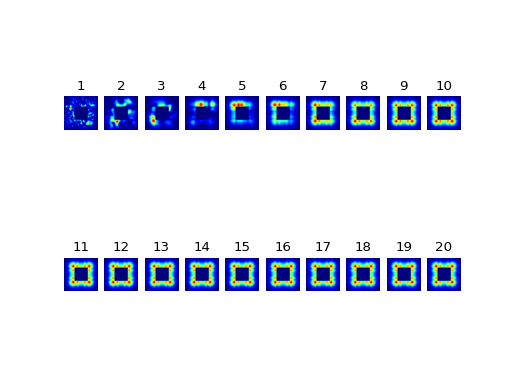(png, hires.png, pdf)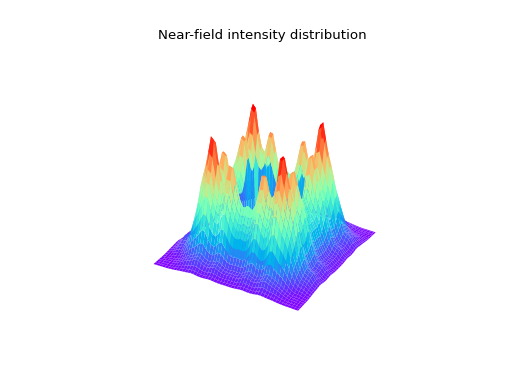(png, hires.png, pdf)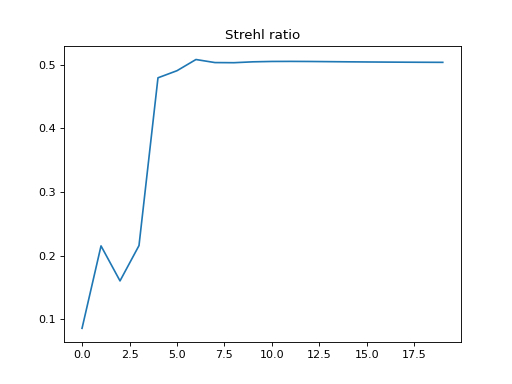(png, hires.png, pdf)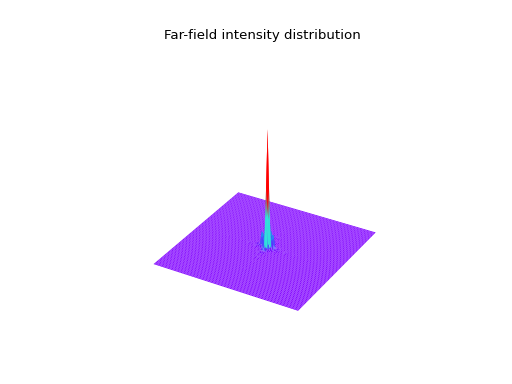(png, hires.png, pdf)

6.5. Phase recovery.¶

6.5.1. Phase recovery using Gerchberg Saxton iteration.¶

#! /usr/bin/python
"""
Phase recovery from two measured intensity distributions using Gerchberg Saxton.

..  :copyright: (c) 2017 by Fred van Goor.
:license: MIT, see License for more details.
"""

import matplotlib.pyplot as plt
import numpy as np
from LightPipes import *
print(LPversion)
#Parameters used for the experiment:
size=11*mm; #The CCD-sensor has an area of size x size (NB LightPipes needs square grids!)
wavelength=632.8*nm; #wavelength of the HeNe laser used
z=2*m; #propagation distance from near to far field
N_iterations=300 #number of iterations

#Read near and far field (at a distance of z=2 m) from disk:
f=open('Inear.prn','r')
f.close()
data = [line.split() for line in lines]
Inear = np.asfarray(data)

f=open('Ifar.prn','r')
f.close()
data = [line.split() for line in lines]
Ifar = np.asfarray(data)

N=len(Inear)
N_new=256;size_new=40*mm;

plt.subplot(3,2,1);plt.imshow(Inear,cmap='jet');
plt.title('Measured Intensity near field'); plt.axis ('off');
plt.subplot(3,2,2);plt.imshow(Ifar,cmap='jet');
plt.title('Measured Intensity far field');plt.axis ('off');

#Define a field with uniform amplitude- (=1) and phase (=0) distribution
#(= plane wave)
F=Begin(size,wavelength,N);

#The iteration:
for k in range(1,100):
print(k)
F=SubIntensity(Ifar,F) #Substitute the measured far field into the field
F=Interpol(size_new,N_new,0,0,0,1,F);#interpolate to a new grid
F=Forvard(-z,F) #Propagate back to the near field
F=Interpol(size,N,0,0,0,1,F) #interpolate to the original grid
F=SubIntensity(Inear,F) #Substitute the measured near field into the field
F=Forvard(z,F) #Propagate to the far field

#The recovered far- and near field and their phase- and intensity
#distributions (phases are unwrapped (i.e. remove multiples of PI)):
Ffar_rec=F;
Ifar_rec=Intensity(0,Ffar_rec); Phase_far_rec=Phase(Ffar_rec);

Phase_far_rec=PhaseUnwrap(Phase_far_rec)
Fnear_rec=Forvard(-z,F);
Inear_rec=Intensity(0,Fnear_rec); Phase_near_rec=Phase(Fnear_rec);

Phase_near_rec=PhaseUnwrap(Phase_near_rec)
#Plot the recovered intensity- and phase distributions:
plt.subplot(3,2,3);plt.imshow(Inear_rec,cmap='jet');
plt.title('Recovered Intensity near field'); plt.axis ('off')
plt.subplot(3,2,4);plt.imshow(Ifar_rec,cmap='jet');
plt.title('Recovered Intensity far field'); plt.axis ('off')
plt.subplot(3,2,5);plt.imshow(Phase_near_rec,cmap='jet');
plt.title('Recovered phase near field');plt.axis ('off')
plt.subplot(3,2,6);plt.imshow(Phase_far_rec,cmap='jet');
plt.title('Recovered phase far field'); plt.axis ('off')

plt.show()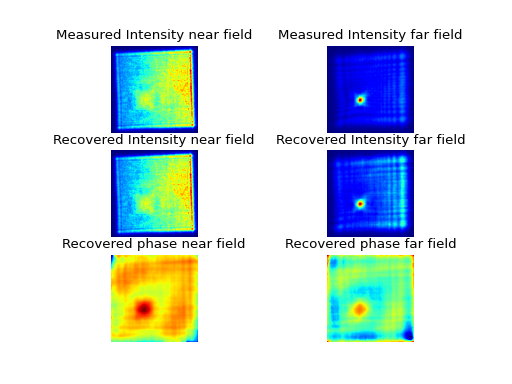6.6. Zernike aberration.¶

Any aberration in a circle can be decomposed over a sum of Zernike polynomials. The Zernike command accepts four arguments: 1. The radial order n 2. The azimuthal order m. 3. The radius, R 4. The amplitude of the aberration.

#!/usr/bin/python
"""
This example demonstrates the Zernike command.
..  :copyright: (c) 2017 by Fred van Goor.
:license: MIT, see License for more details.
"""

from LightPipes import *
import matplotlib.pyplot as plt
import math
import numpy as np

wavelength=500*nm
size=2.0*mm
N=200
A=wavelength/(2*math.pi)

plt.figure(figsize=(15,8))
for Noll in range (1,22):
(nz,mz)=noll_to_zern(Noll)
S=ZernikeName(Noll)
F=Begin(size,wavelength,N)
F=Zernike(nz,mz,size/2,A,F)
F=CircAperture(size/2,0,0,F)
Phi=Phase(F)
plt.subplot(3,7,Noll)
plt.imshow(Phi, cmap='jet')
s=repr(Noll) + '  ' + ' $Z^{'+repr(mz)+'}_{'+repr(nz)+'}$' + '\n' + S
plt.title(s, fontsize=9);plt.axis('off')
plt.show()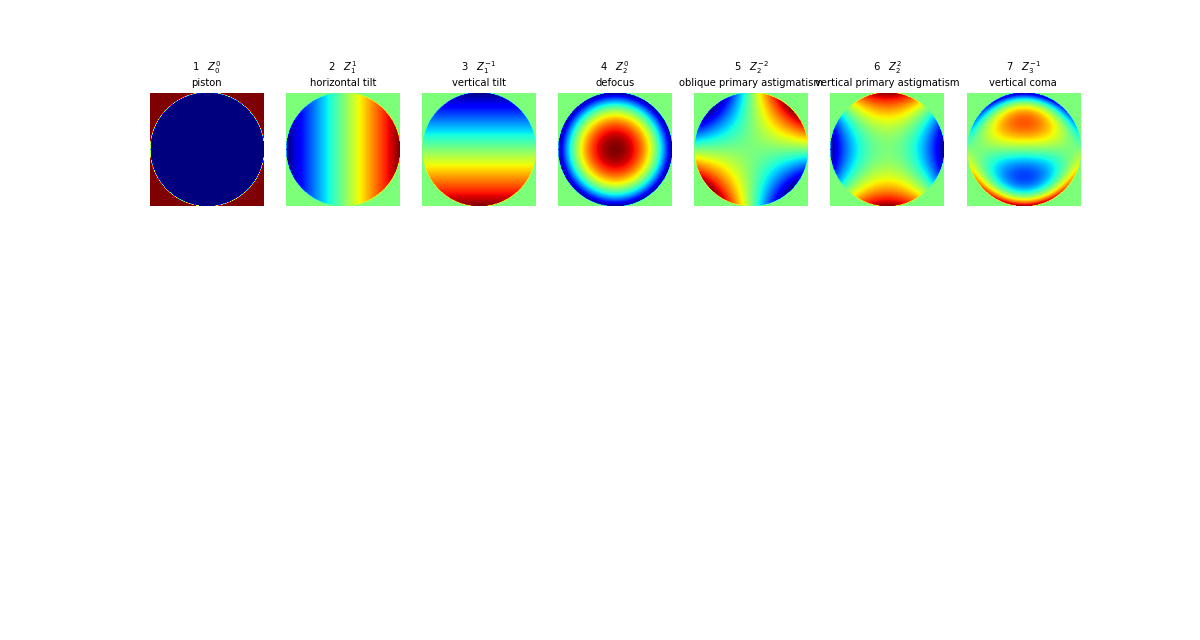6.6.1. Radial shear interferometer.¶

#!/usr/bin/python
"""
This example demonstrates a radial shear interferometer.
Only a 'bare-bone' model, so no propagation and diffraction, is considered.
..  :copyright: (c) 2017 by Fred van Goor.
:license: MIT, see License for more details.
"""

from LightPipes import *
import matplotlib.pyplot as plt
import math

pi=3.1415

wavelength=500*nm
size=40.0*mm
N=200
R=10*mm
nz=10
mz=4
Rz=10*mm

Az=wavelength/(2*pi)

Rbs=0.5
M=1.5

F=Begin(size,wavelength,N)

F=Zernike(nz,mz,Rz,Az,F)
F=CircAperture(R,0,0,F)
phi=Phase(F)
fig=plt.figure(figsize=(8,8))
fig.suptitle('radial shear interferometer',fontsize=20, color='red')
plt.subplot(2,1,1)
plt.imshow(phi,cmap='jet');plt.axis('off');plt.axis('equal')
plt.title('phase distribution input beam')
F1=IntAttenuator(Rbs,F)
F2=IntAttenuator(1-Rbs,F)

F1=Interpol(size,N,0,0,0,M,F1)
F=BeamMix(F1,F2)
I=Intensity(2,F)
plt.subplot(2,1,2)
plt.imshow(I,cmap='jet')
plt.axis('off');plt.axis('equal')
plt.title('intensity distribution output beam')

plt.show()6.7. Propagation in a lens-like, absorptive medium.¶

In this example we model the propagation of a Gaussian beam in a lens-like waveguide. The profile of the refractive index is chosen such, that the beam preserves approximately its diameter in the waveguide (we use the fundamental mode). We’ll consider the propagation of an axial mode, tilted with respect to the waveguide axis and a non-axial mode.

we use the approximation for the profile of the refractive coefficient, $$n'=n-i\kappa$$ in the form: $$n(r)^2=n_0^2-n_0n_1r^2$$. It is a well-known fact  that the half-width of the fundamental Gaussian mode of a lens-like waveguide is defined as: $$w_0^2=\frac{2}{k(n_0n_1)^{1/2}}$$ , with $$k=\frac{2 \pi}{\lambda}$$. For a waveguide of $$1 \times 1 mm, n_0=1.5, n_1=400 m^{-2}, \kappa = 1.0$$ and $$\lambda = 1 \mu m$$, the Gaussian mode has a diameter of $$226 \mu m$$ . A tilt in the x-direction causes reflections in the waveguide as demonstrated in the next example of the propagation of a tilted Gaussian beam through the waveguide.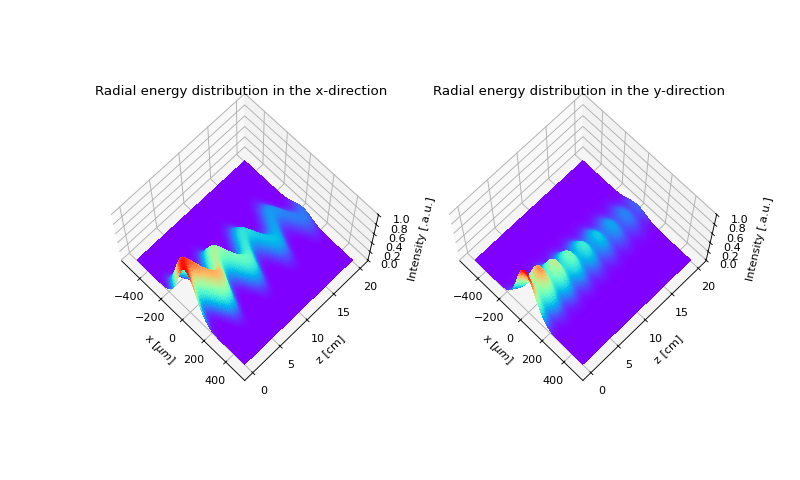Propagation of a tilted Gaussian beam in a lens-like, absorptive medium.

  Marcuse, Light Transmission Optics, Van Nostrand Reinhold, 267-280, (1972).

6.8. Fourier optics.¶

6.8.1. Pattern recognition.¶

In this example we demonstrate the recognition of objects using Fourier optics. The light, originating from a collection of objects, is focused with a positive lens. A second lens is positioned with its primary focal point in the focus of the first lens. When we place a screen behind the second lens at its secondary focus an inverted image of the collection of objects is projected on the screen, as shown in figure 1. The object to be recognized is present once or several times in the collection. As objects we choose transparencies with the characters A, B, and C.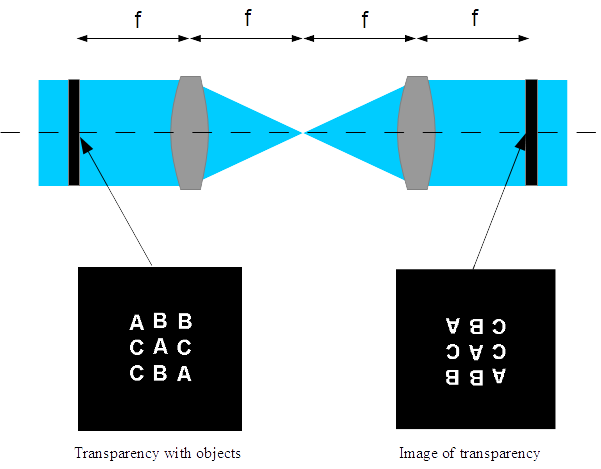Fig. 1 Imaging a transparency with objects

Each object will contribute to the phase distribution in the secondary focus of the first lens. If the fluctuations in the wavefront coming from one of the objects is compensated by a phase plate prepared for that sort of object, see figure 2, placed in the focus, the beam coming from those objects will propagate as a diverging spherical wave to the second lens and will be focused in a diffraction limited point on the screen. The position of that point will indicate the presence and the position of the object. This will only be the case when the object, for which the phase plate was positioned in the focus, is actually present in the collection.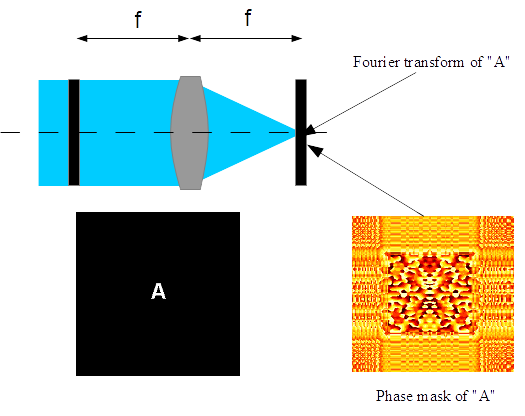Fig. 2 Making a phase mask of the Fourier transform of an objectFig. 3 Placing the phase mask in the focus of the first lens.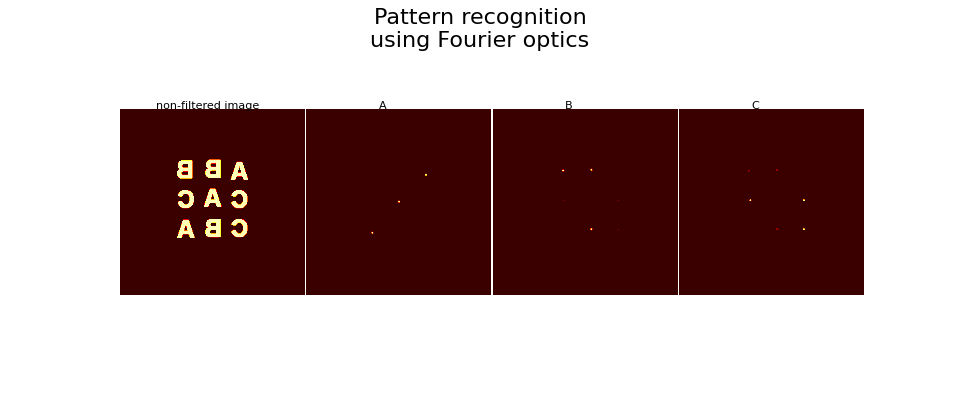Fig. 4 Results of the pattern recognition simulation.